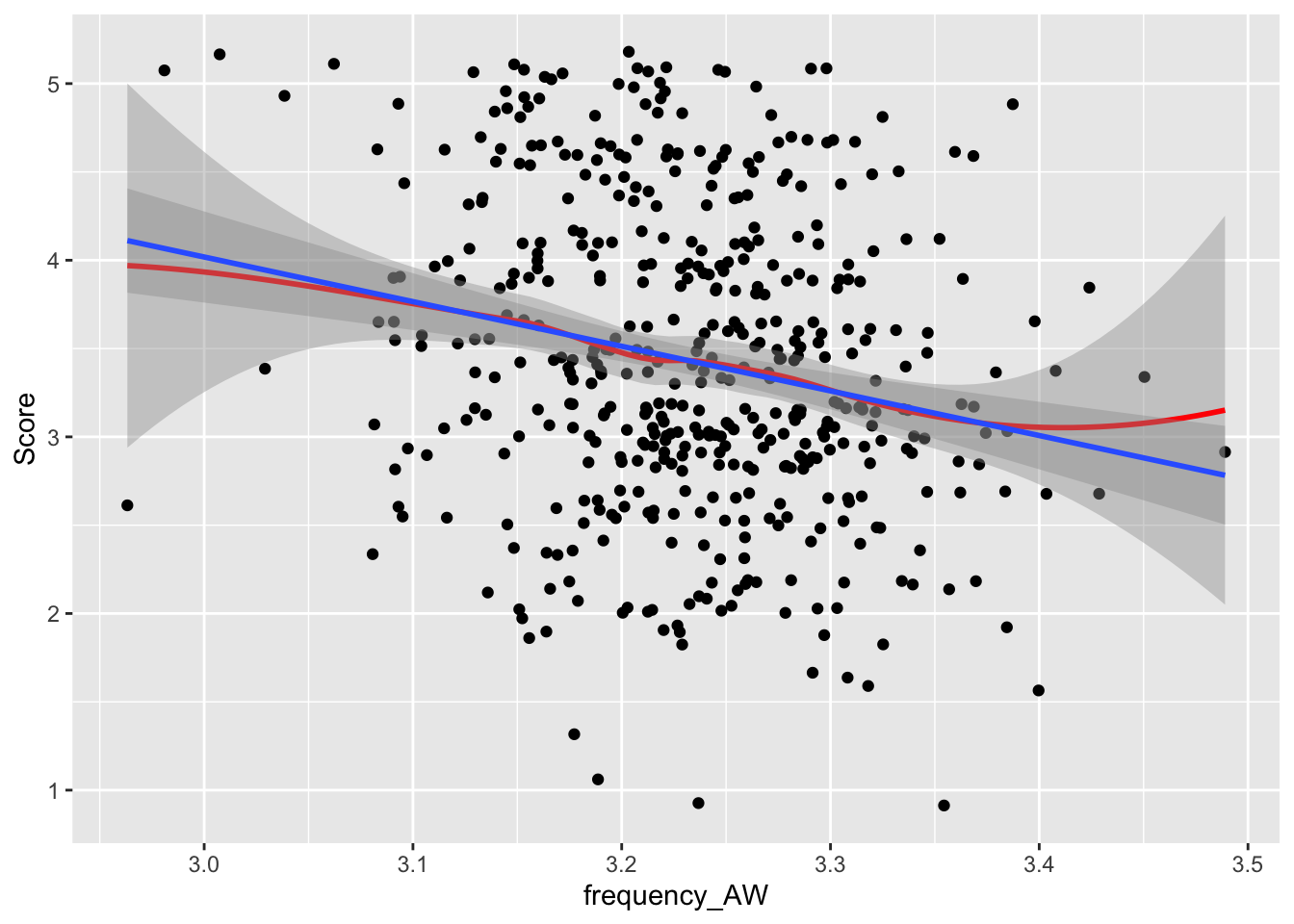## Multiple Regresion: Using multiple independent variables to predict dependent variable values

Often, particular constructs (such as linguistic proficiency) are multifaceted and are best measured using multiple measures. In such cases, we can use multiple regression to predict a dependent variable (such as linguistic proficiency) using multiple independent variables (such as features of lexical sophistication and syntactic complexity). This tutorial builds on the previous two tutorials on Correlation and Linear Regression, so be sure to check those out. In this tutorial, we are going to predict holistic writing quality scores (Score) using a number of linguistic features related to frequency and an index of syntactic complexity (mean length of clause).

### Assumptions

The assumptions for multiple regression are very similar to those of Pearson correlations and (single) linear regression. We do, however, add one important assumption: (non) multicollinearity.

The main assumptions of (single) linear regression are:

• The variables must be continuous (see other tests for ordinal or categorical data)

• The variables must have a linear relationship with the dependent variable

• There are no outliers (or there are only minimal outliers in large samples)

• The residuals must be normally distributed

• The predictor variables are not strongly correlated with each other (this is referred to as multicollinearity)

#### Assumptions 1-3: The variables must be continuous, there must be a linear relationship between each independent (predictor) variable and the dependent variable, and there are no (minimal) outliers.

First, we will load our data, then we will make a series of scatterplots. Note that “Score” represents holistic writing quality scores that range from 1-5 in .5 point increments. For the purposes of this tutorial, we will consider “Score” to be a continuous variable. Also note that we will use geom_jitter() instead of geom_point() to help us visualize the linearity.

``````mr_data <- read.csv("data/multiple_regression_sample.csv", header = TRUE)
summary(mr_data)``````
``````##      Score        frequency_AW    frequency_CW    frequency_FW
##  Min.   :1.000   Min.   :2.963   Min.   :2.232   Min.   :3.598
##  1st Qu.:3.000   1st Qu.:3.187   1st Qu.:2.656   1st Qu.:3.827
##  Median :3.500   Median :3.237   Median :2.726   Median :3.903
##  Mean   :3.427   Mean   :3.234   Mean   :2.723   Mean   :3.902
##  3rd Qu.:4.000   3rd Qu.:3.284   3rd Qu.:2.789   3rd Qu.:3.975
##  Max.   :5.000   Max.   :3.489   Max.   :3.095   Max.   :4.235
##  bigram_frequency      MLC
##  Min.   :1.240    Min.   : 5.769
##  1st Qu.:1.440    1st Qu.: 8.357
##  Median :1.500    Median : 9.584
##  Mean   :1.500    Mean   : 9.730
##  3rd Qu.:1.559    3rd Qu.:10.794
##  Max.   :1.755    Max.   :20.381``````

Frequency_AW

``````library(ggplot2)
ggplot(mr_data, aes(x = frequency_AW , y=Score )) +
geom_jitter() +
geom_smooth(method = "loess",color = "red") + #this is a line of best fit based on a moving average
geom_smooth(method = "lm") #this is a line of best fit based on the enture dataset``````Frequency_CW

``````ggplot(mr_data, aes(x = frequency_CW , y=Score )) +
geom_jitter() +
geom_smooth(method = "loess",color = "red") + #this is a line of best fit based on a moving average
geom_smooth(method = "lm") #this is a line of best fit based on the enture dataset``````